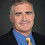## Wednesday, January 09, 2019

### Search versus Decision

Shockingly I've never done a post on search versus decision, one of the more interesting dualities in complexity. In short: Decision: Is there a needle in the haystack? Search: Find the needle.

In Satisfiability, or any other NP-complete problem, the two problems are essentially equivalent. If you can decided SAT you can find a solution (good homework problem) or even the best solution. Often people mix up the two, where people say finding the shortest Traveling Salesman Tour is NP-complete, usually without getting into too much trouble.

Decision is always at least as easy as search: If you have a solution you know there is one. What about the other direction? We can't actually prove search is hard without separating P and NP, but we have our conjectures.

Sometimes both are easy. We can easily find the maximum weighted matching.

Sometimes decision is easy and search is supposedly hard: Composite Numbers. The search version is factoring.

Sometimes decision is trivial (i.e. they always exist) and search is still hard. Nash Equilibria. Ramsey Graphs.

Often we ask whether search reduces to decision? If you have some oracle (magic black box) that answered decision questions, can you solve the search problem efficiently? SAT has this property, as does Matching (for trivial reasons). Nash Equilibrium and Composite Numbers likely don't.

Graph Isomorphism does, i.e., given an oracle for graph isomorphism you can find the isomorphism (another good homework problem).

There's also an interesting non-adaptive version. Given a SAT formula can you find an assignment with questions to a SAT oracle that all have to be asked at the same time?

Here we get a probable yes. If the formula has one solution you can find it by asking for each bit of the solution. Randomly you can reduce SAT to several formulas, one of which is likely to have a single assignment that is also an assignment of the original formula. With standard hardness assumptions you can eliminate the randomness.

Is the same true for graph isomorphism? I think that's still open.

1.I think "If you have some oracle (magic black box) that answered search questions, can you solve the decision problem efficiently?" should be "If you have some oracle (magic black box) that answered decision problem, can you solve the search questions efficiently?"

1.Thanks. Fixed.

2.One step in reducing Graph Isomorphism search to non-adaptive Graph Isomorphism decision: For each vertex u in G and v in H, make a new pair of graphs, G' and H', where G' is the same a G except with a newly added large clique attached to u, and H' is the same as H except with a newly added large clique attached to v. Then G' and H' will be isomorphic iff there is an isomorhpism between G and H that maps u to v. Unfortunately, there may be many isomorphisms between G and H, so these queries do not allow us to non-adaptively find an isomorphism between G and H.

3.Only two notes:

* the factoring problem seems to revert to normality if you ask: "given an integer N does it have more than two factors?"

* a search problem is equivalent to a decision problem of the form "Given an instance x and two certificates a,b; does there exist a solution between a and b"? (x,a,b can be simple integers, provided that an efficient decode/encode enumeration of the instances and certificates exists).

So (perhaps) the focus could be switched ("normalized") to the difference between "Does there exist a solution between a and b?" and "Does there exist a solution?" (both decision problems)

4.Is it known if P \neq NP, then search is harder than decision?

1.I don't think this is known assuming only P neq NP, but Bellare & Goldwasser (SIAM J. Comput., 1994) showed that if EE neq NEE, then there are languages in NP for which search is harder than decision.

5.I've invented Quaternionic Optimization(Programming)

http://vixra.org/abs/1812.0174

6.Search-to-decision for *group* isomorphism remains an interesting open problem. Although search-to-decision for graph isomorphism has been known for a long time, and group isomorphism (in the multiplication/Cayley table model) is easier than graph isomorphism, we still don't know how to do a search-to-decision reduction for groups.

7.Hey Josh,

I couldn't find an algorithm for group isomorphism. Can you provide a link?

We might consider a group to be a set of ordered triples following the usual group rules (associativity, closure, unique identity, unique inverses, and so on), then think of the isomorphism as a constraint satisfaction instance on said triples. CSPs have search-to-decision reductions like SAT, right?

Hope all is well,
NPS

8.Hmm. What I wrote isn't quite right, though admittedly late at night for me. A decider for group isomorphism obviously isn't a decider for CSP.